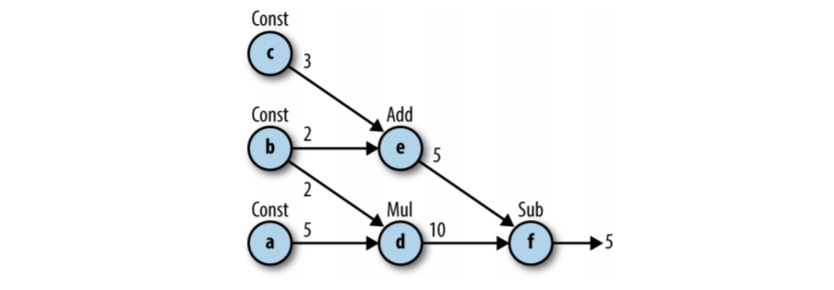# Basic TensorFlow Building Blocks

The following is an abbreviated look at Chapter 3 of Learning TensorFlow.

### Workflow

Working in TensorFlow boils down to two simple steps

2. Run it in a session

Say you wanted to use TensorFlow to implement the following.

from IPython.display import Image

Image('images/graph.png')Arriving at our final value of 5 requires a few intermediate values along the way. We could express this in pure python with the following:

a = 5
b = 2
c = 3
d = a * b
e = b + c
f = d - e
print(f)
5


The key difference between this approach and how TensorFlow is organized is that whereas all of this execution is actually happening line by line, when we construct our network, nothing actually gets exectuted. For example:

import tensorflow as tf

a = tf.constant(5)
b = tf.constant(2)
c = tf.constant(3)

d = tf.multiply(a, b)

f = tf.subtract(d, e)

Then, once we have our execution graph, we can run everything by spinning up a Session object. As a best practice, we’ll use a context manager.

with tf.Session() as sess:
result = sess.run(f)

print(result)
5


A quick note on this– f, our result variable, depended on everything before it (see the execution graph above). As a result, TensorFlow went ahead and executed each node in the graph (a process called fetching). If, however, we didn’t need any node in the graph, it would happily skip over it and save us resources/time.

### Variables and Placeholders

As we saw above, we constructed our graph by instantiating some nodes and then doing things to them. In the example, we made constants, but there are other types of nodes worth using.

• tf.Variable, the trainable parameters of our model, modified as a result of training
• tf.placeholder, used to pass input data

#### Variables

Unlike tf.constants which are immutable, these objects can change as a result of execution, but also maintain state iteration-to-iteration.

Furthermore, they must be explicitly instantiated by running tf.global_variables_initializer() at the beginning of your tf.Session().

init_vals = [1, 2, 3]
var = tf.Variable(init_vals)

init = tf.global_variables_initializer()

with tf.Session() as sess:
sess.run(init)
print(sess.run(var))
[1 2 3]


#### Placeholders

On the other hand, we’ll often want to pass data into a model for training/scoring purposes. If a regression is a big y = mX + b problem, we need a way to pass in the X.

Thus we use tf.placeholder(), passing in a datatype and an expected shape. The shape variable can be a tuple of fixed size, but if we pass None as any of the dimensions, we allow for arbitrarily many observations (often on the number-of-records-level).

The following are all valid instantiations.

tf.placeholder(tf.float32, shape=(1, 10))
tf.placeholder(tf.float64, shape=(None, 100))
tf.placeholder(tf.bool, shape=(None));

To actually use the placeholder, though, you must pass the feed_dict argument to your Session().run() call.

As a quick example, say you wanted, for some reason, to normalize a list of floats relative to all of the values in the list… using TensorFlow.

vals = tf.placeholder(tf.float64, shape=(None,))

mean, stdev = tf.nn.moments(vals, axes=0)

normed = (vals - mean) / stdev
input_data = [1, 2, 3, 4, 5, 6, 7]
with tf.Session() as sess:
normed_vals = sess.run(normed,
feed_dict={vals: input_data})

print(normed_vals)
[-0.75 -0.5  -0.25  0.    0.25  0.5   0.75]


#### A note on datatypes

If you’ve got incompatible types between two tensors, the whole execution will error out.

a = tf.constant(1.0)
b = tf.constant(2)

try:
c = a + b
except TypeError:
print("Whoops")

with tf.Session() as sess:
sess.run(c)
Whoops


But we can easily fix this using tf.cast()

a = tf.constant(1.0)
b = tf.constant(2)

try:
a = tf.cast(a, tf.int32)
c = a + b
except TypeError:
print("Whoops")

with tf.Session() as sess:
print(sess.run(c))
3